# Electric Lines of Force

If a free to move unit positive charge is placed in an electric field, it will experience a force due to which the unit positive charge will move along a particular path. The unit positive charge can be placed at different positions in the field and the unit positive charge will follow different paths when it is placed in different positions. The paths, along which the unit positive charge will move due to electrostatic force in the field are called electric lines of force.

We consider the direction of electric lines of force as the direction of the movement of unit positive charge in the field. We represent the direction of lines of force with arrowheads.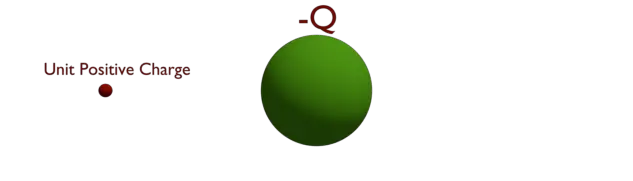Let us take a negative charge of – Q coulomb. Also, let us place a unit positive charge at a point in the field of charge – Q.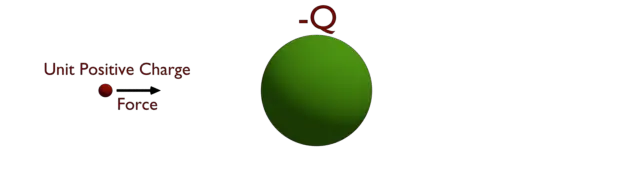According to Coulomb’s law, the unit positive charge will experience an attraction force, towards the center of the said negative charge.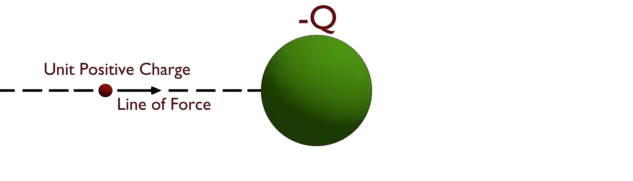If the unit positive charge is free to move, that will move towards the charge – Q, along the straight line joining the center of unit positive charge and the center of said negative charge.

The line along which the unit positive charge moves towards the said negative charge, is defined as an electric line of force. If we placed the unit positive charge anywhere else in the field, the line of force was created along that new point.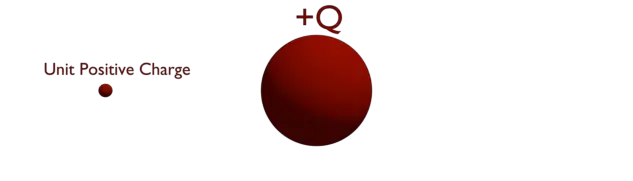Now, let us take a positive charge of + Q coulomb. We place a unit positive charge at a point in the field.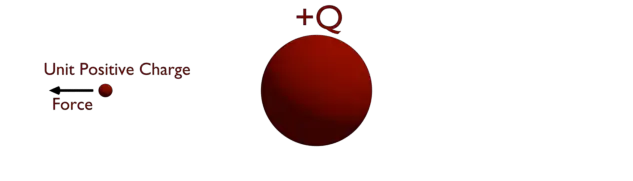According to Coulomb’s law, the unit positive charge will experience a repulsive force.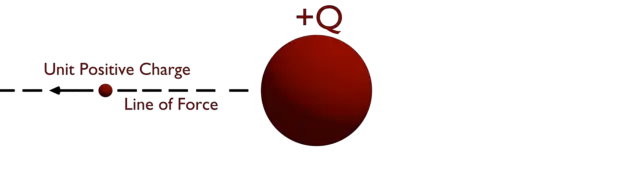As the unit positive charge is free to move, that will move away along the straight line joining the center of unit positive charge and the said positive charge. The line along which the unit positive charge moves away from the said positive charge is an electric line of force. Similarly, if we placed the unit positive charge anywhere else in the field, the line of force was created along that new point.

Since, we can place a unit positive charge, at infinite number of points in the electric field, theoretically there will be infinite number of lines of force created in the field of an electric charge. But mathematically, to represent the influence of electric charge in the field, the total number of lines of force emanated from an electric charge is taken as equal to the value of electric charge measured in coulomb. That means a Q Coulomb charge emanates Q number of lines of force surrounding it.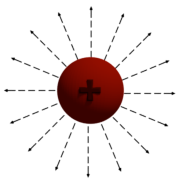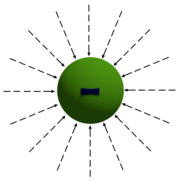Now, let us place one positively charged object of + Q Coulomb and one negatively charged object of – Q Coulomb side by side as shown, below. Let us place a unit positive charge at any point in between these two charged objects. The unit positive charge will experience an attraction force by the negatively charged object and a repulsion force by the positively charged object. Due to influence attraction and repulsion force, the unit positive charge will move along a curved line from the center of the + Q charged object to the center of – Q charged object. Considering other lines of forces, we can draw the electric field like below.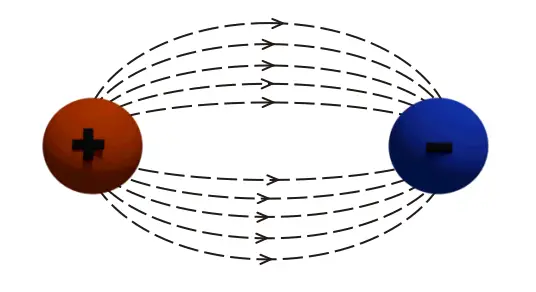Now, let us place two positively charged objects of + Q Coulomb side by side as shown, as shown below. Let us place a unit positive charge at any point on any of the charged objects. The unit positive charge will experience a repulsion force by + Q charge on which the unit positive charge is placed. The unit positive charge also experiences another repulsive force by the nearby + Q charge. Due to the influence of these two repulsion forces from both sides, the unit positive charge will move away from both + Q charges, as shown, as shown below. Considering other lines of forces, we can draw the electric field like below.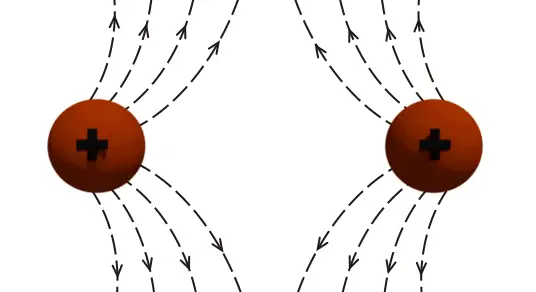## Properties of Electric Lines of Force

1. Each electric lines of force is conceptually imagined it does not have any physical existence.
2. Total number of lines of force emanated from a charge body is equal to the charge of the body measured in Coulomb.
3. Each electric lines of force is originated from positive charge and terminated to the negative charge.
4. A tangent drawn at any point on an electric line of force, indicates the direction electric field at that point in the field.
5. Each electric line of force emanates normally from the surface of the charge body.
6. Electric lines of force can only contract longitudinally.
7. Electric lines of force can expand laterally.
8. No two electric lines of force cross each other.
9. The electric lines of force in same direction repel each other.
10. The electric lines of force in opposite direction attract each other.
11. No lines of force can exists inside the conductor.

Want To Learn Faster? 🎓
Get electrical articles delivered to your inbox every week.
No credit card required—it’s 100% free.